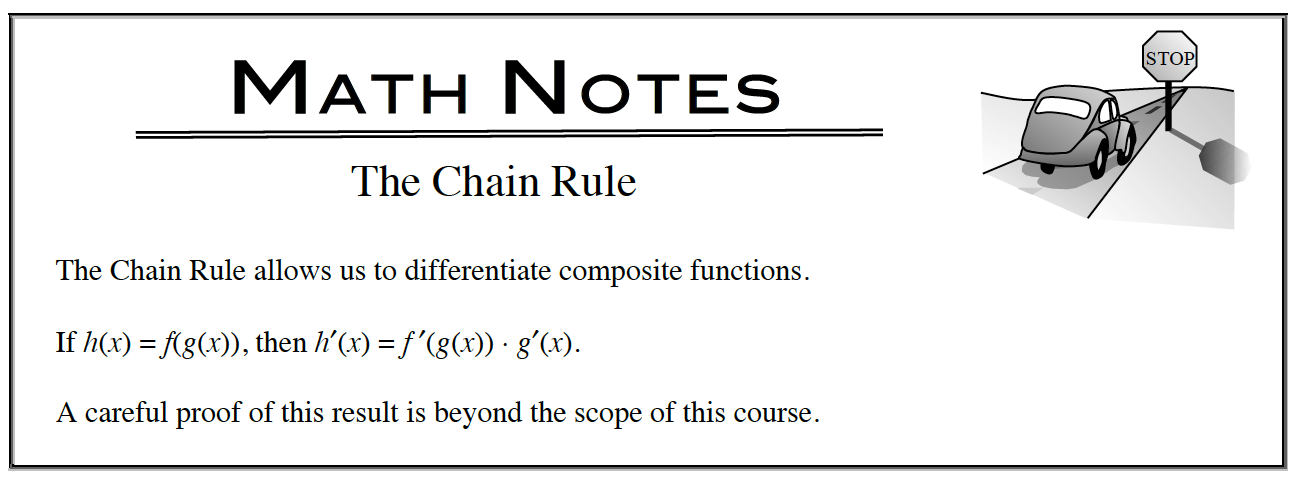### Home > APCALC > Chapter 5 > Lesson 5.2.2 > Problem5-74

5-74.

Chris found the derivative of $(2x + 5)^2$ as shown below. Homework Help ✎
$\frac { d } { d x }((2x + 5)^2)$ $=\frac { d } { d x }(4x^2 + 20x + 25) = 8x + 20$

To check his solution, he tried applying the Power Rule:
$\frac { d } { d x }((2x + 5)^2) = 2(2x + 5) = 4x + 10$

His solutions did not match! Which is the correct derivative, and why?